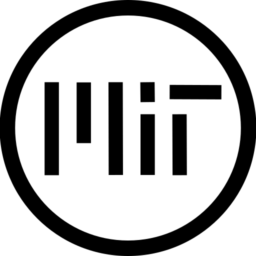# Colorful Outline

The shader was originally made by /u/MinRaws over 3 years ago here

I basically just added some extra colour features and a few tweaks.

Colouring either uses two colours that’ll fade into each other or you can use a texture.

A gradient texture (like in the post) is recommend, but you can use any texture if you really wanted.

You can flip the colours around so you can get either a horizontal or vertical fade.

If anyone has any suggestions for improvements that would be greatly appreciated!

``````shader_type canvas_item;

uniform int intensity = 50;
uniform float precision : hint_range(0,0.02);
uniform bool flipColors;		//Flip coloring 90 degrees.

//If not using a texture, will blend between these two colors
uniform vec4 outline_color : hint_color;
uniform vec4 outline_color_2 : hint_color;

uniform bool use_outline_uv;	//Use the outline_uv for coloring or not. Recomended not, but sometimes might be good.

uniform bool useTexture;		//Use a texture for the coloring
uniform sampler2D outlineTexture;	//This is the texture that will be used for coloring. Recomended to use a gradient texture, but I guess anything else works.

varying vec2 o;
varying vec2 f;

void vertex()
{
//Expands the vertexes so we have space to draw the outline if we were on the edge.
o = VERTEX;
vec2 uv = (UV - 0.5);
VERTEX += uv * float(intensity);
f = VERTEX;
}

void fragment()
{
ivec2 t = textureSize(TEXTURE, 0);
vec2 regular_uv;
regular_uv.x = UV.x + (f.x - o.x)/float(t.x);
regular_uv.y = UV.y + (f.y - o.y)/float(t.y);

vec4 regular_color = texture(TEXTURE, regular_uv);

if((regular_uv.x < 0.0 || regular_uv.x > 1.0) || (regular_uv.y < 0.0 || regular_uv.y > 1.0) || regular_color.a <=0.25){
regular_color = vec4(0.0);
}

vec2 ps = TEXTURE_PIXEL_SIZE * float(intensity) * precision;

vec4 final_color = regular_color;
if (regular_color.a <= 0.0)
{
for(int x = -1; x <= 1; x += 1){
for(int y = -1; y <= 1; y += 1){

//Get the X and Y offset from this
if (x==0 && y==0)
continue;

vec2 outline_uv = regular_uv + vec2(float(x) * ps.x, float(y) * ps.y);

//Sample here, if we are out of bounds then fail
vec4 outline_sample = texture(TEXTURE, outline_uv);
if((outline_uv.x < 0.0 || outline_uv.x > 1.0) || (outline_uv.y < 0.0 || outline_uv.y > 1.0)){
//We aren't a real color
outline_sample = vec4(0);
}

vec2 final_uv = use_outline_uv ? outline_uv : UV;	//get the UV we will be using, controlled via use_outline_uv

//Is our sample empty? Is there something nearby?
if(outline_sample.a > final_color.a){
if(!useTexture)	//If we're not using a texture
{
final_color = mix(outline_color, outline_color_2, flipColors ? final_uv.y : final_uv.x);
}
else
{
vec2 uv = flipColors ? vec2(final_uv.y, final_uv.x) : final_uv;
vec4 outline = texture(outlineTexture, uv);

final_color = outline;
}
}
}
}
}
COLOR = final_color;
}``````
###### Tags
Color, colour, outlineThe shader code and all code snippets in this post are under MIT license and can be used freely. Images and videos, and assets depicted in those, do not fall under this license. For more info, see our License terms.

### Smooth outline 2DInline FeedbacksGammaGames
4 months ago

The texture outline feature is really powerful, I did it with some tiling pattern and it came out really interestingly. I wish we could set a tint for the texture, it would make some cool picture frames.

Edit: Also I don’t think it works on atlas textures, that’s a shame

Last edited 4 months ago by GammaGameslyghtkruz
7 hours ago

This works for any items that don’t have to flip. When I flip horizontal it distorts the shape. I’m assuming it has to do with the way it does the for loop, but I’m not sure.